Stats
Challenges
Controls
Help
Click the Get hint button below and then click the cell you want a hint for.
Difficulty:
Easy Medium Hard
Size:
Random 3x3 4x4 5x5
GO
Do you want to:
-OR-
Kakuro is a crossword-like puzzle game that uses numbers and math instead of words.
The Basics
• The value for a cell must be 1-9
• Duplicate numbers are not allowed within a clue (4=1+3, 4≠2+2)
• Each puzzle has a unique solution that can be solved without guesswork
Puzzle Layout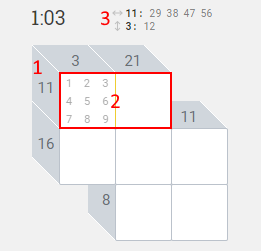1. The number 11 is a clue
3. Possible combinations for the horizontal (11) and vertical (3) clues
Solving a Simple Puzzle
Let's solve a simple 3x3 puzzle step by step. Keep in mind that there are many different ways to arrive at the same unique solution to a puzzle.
Step 1: Since the only combination for 23 into three cells is 6+8+9 we put 6, 8 and 9 in each answer cell for 23.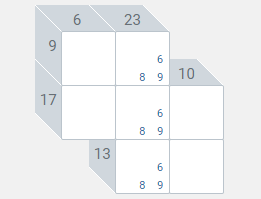Step 2: For 9 across we remove the 9 in the second cell since 0+9=9 contains a zero. We then put 1 and 3 in the first cell (1+8=9 and 3+6=9).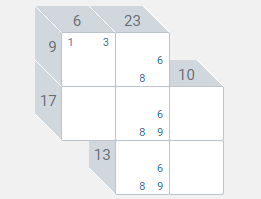Step 3: For 6 down we remove the 3 in the first cell since 3+3=6 contains duplicates. That leaves us with 1 in the first cell so we put 5 in the second cell (1+5=6).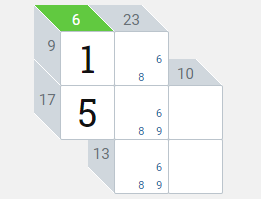Step 4: For 9 across we put 8 in the second cell (1+8=9). We then remove the 8 from the second and third cells of 23 since duplicates are not allowed.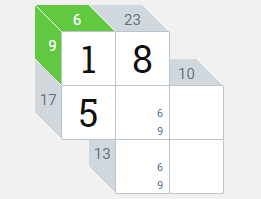Step 5: For 17 across we know the only valid combination is 5+9+3 since 5+6+6 contains duplicates.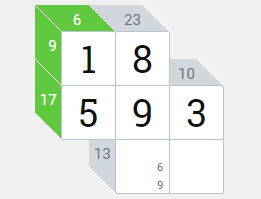Step 6: We can now finish the puzzle (8+9+6=23, 6+7=13 and 3+7=10).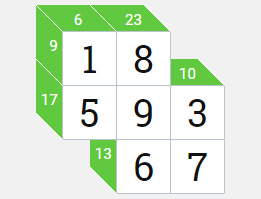Tips
Tip 1
Start with clues that have only one combination such as 3=1+2, 4=1+3, 16=7+9, 17=8+9, 6=1+2+3, and so on.
Tip 2
Take advantage of the possible combinations area located above the puzzle: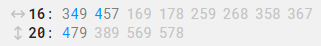This area shows all possible combinations for the vertical and horizontal clues for the current cell. Numbers already entered in the answer cells for a clue will be blue. Combinations no longer valid are grayed out. Looking at the example above, we can determine the remaining two cells for the vertical clue (20) will be 7+9.
Tip 3
Use fast select and drag to improve your speed.

Fast select refers to selecting a single number regardless of how many numbers are currently selected within a cell. To fast select, touch and hold a number for 1 second. When using a physical keyboard, press and hold a number key for 1 second.

Use drag to select multiple numbers at once within a cell. Simply touch a number and drag your finger over additional numbers.
Tip 4
Consider creating a KakuroGame account. Having an account allows you to sync data (stats and in-progress puzzles) between devices. For example, you can start a puzzle on your phone and finish it on your computer on our website ().